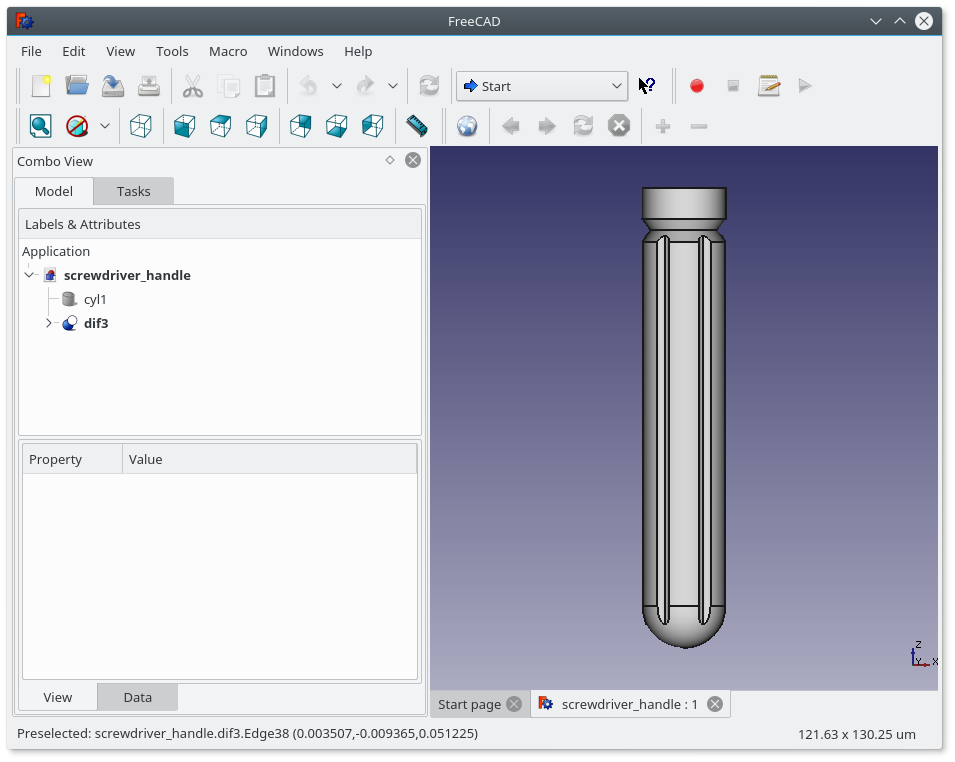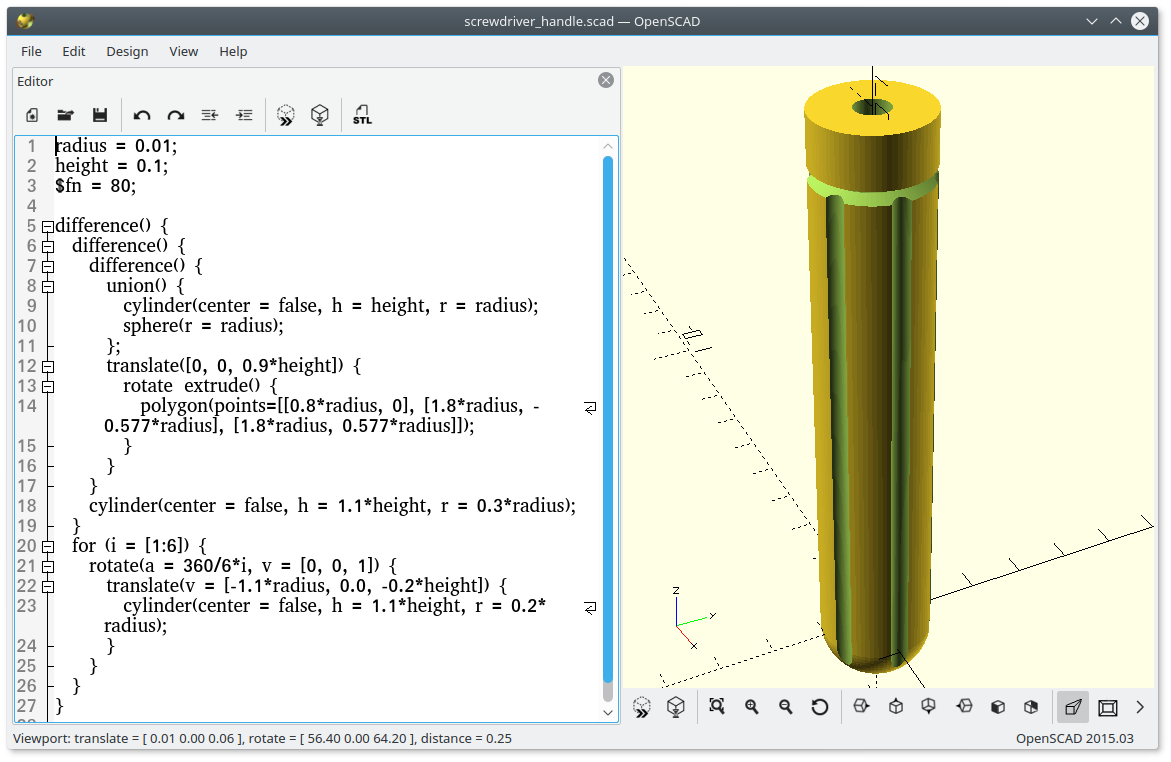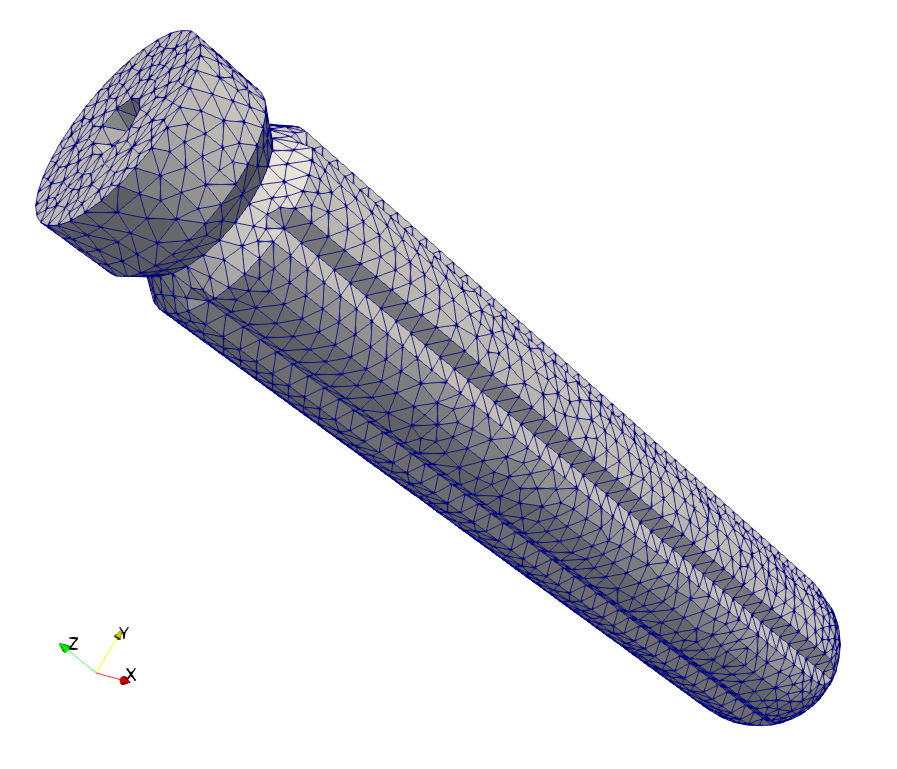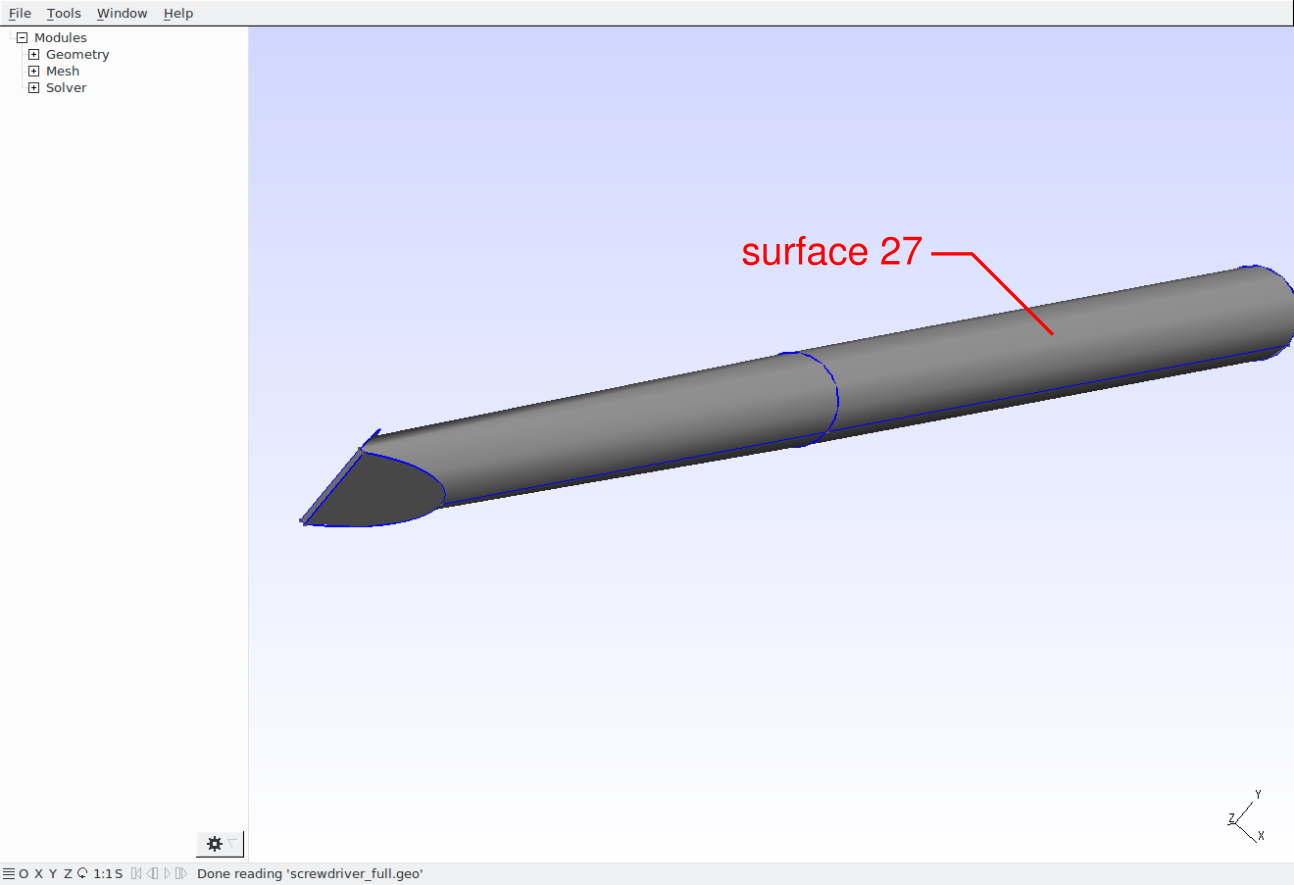## Introduction¶

There are several open source tools for preparing 2D and 3D finite element meshes like Salome, FreeCAD, Gmsh, Netgen, etc. Most of them are GUI based geometrical modeling and meshing environments/tools but they also usually allow using their libraries in user scripts. Some of the above mentioned tools are handy for solid modeling, some of them are great for meshing. This tutorial shows how to combine solid geometry modeling functions provided by FreeCAD or OpenSCAD with meshing functions of Gmsh.

The collaboration of modeling, meshing and conversion tools and the workflow are illustrated in the following scheme.Functionalities of FreeCAD are accessible to Python and can be used to define geometrical models in simple Python scripts. There is a tutorial related to Python scripting in FreeCAD.

The first step in creating a Python script is to set up a path to the FreeCAD libraries and import all required modules:

 ```1 2 3 4 5 6 7 8``` ```import sys FREECADPATH = '/usr/lib/freecad/lib/' sys.path.append(FREECADPATH) from FreeCAD import Base, newDocument import Part import Draft import ProfileLib.RegularPolygon as Poly ```

Now, a new empty FreeCAD document can be defined as:

```doc = newDocument()
```

All new objects describing the geometry will be added to this document.

In the following lines a geometrical model of a screwdriver handle will be created. Let’s start by defining a sphere and a cylinder and join these objects into the one called `uni`:

 ``` 1 2 3 4 5 6 7 8 9 10 11 12``` ```radius = 0.01 height = 0.1 cyl = doc.addObject("Part::Cylinder", "cyl") cyl.Radius = radius cyl.Height = height sph = doc.addObject("Part::Sphere", "sph") sph.Radius = radius uni = doc.addObject("Part::MultiFuse", "uni") uni.Shapes = [cyl, sph] ```

Create a polygon, revolve it around the z-axis to create a solid and use the result as the cutting tool applied to `uni` object:

 ``` 1 2 3 4 5 6 7 8 9 10 11 12 13 14 15``` ```ske = doc.addObject('Sketcher::SketchObject', 'Sketch') ske.Placement = Base.Placement(Base.Vector(0, 0, 0), Base.Rotation(-0.707107, 0, 0, -0.707107)) Poly.makeRegularPolygon('Sketch', 5, Base.Vector(-1.2 * radius, 0.9 * height, 0), Base.Vector(-0.8 * radius, 0.9 * height, 0)) cut = doc.addObject("PartDesign::Revolution", "Revolution") cut.Sketch = ske cut.ReferenceAxis = (ske, ['V_Axis']) cut.Angle = 360.0 dif = doc.addObject("Part::Cut", "dif") dif.Base = uni dif.Tool = cut ```

Create a cylinder, make a polar array of the cylinder objects and subtract it from the previous result:

 ``` 1 2 3 4 5 6 7 8 9 10 11 12 13``` ```cyl1 = doc.addObject("Part::Cylinder", "cyl1") cyl1.Radius = 0.2 * radius cyl1.Height = 1.1 * height cyl1.Placement = Base.Placement(Base.Vector(-1.1 * radius, 0, -0.2 * height), Base.Rotation(0, 0, 0, 1)) arr = Draft.makeArray(cyl1, Base.Vector(1, 0, 0), Base.Vector(0, 1, 0), 2, 2) arr.ArrayType = "polar" arr.NumberPolar = 6 dif2 = doc.addObject("Part::Cut", "dif2") dif2.Base = dif dif2.Tool = arr ```

Create a middle hole for the screwdriver metal part:

 ```1 2 3 4 5 6 7``` ```cyl2 = doc.addObject("Part::Cylinder", "cyl2") cyl2.Radius = 0.3 * radius cyl2.Height = height dif3 = doc.addObject("Part::Cut", "dif3") dif3.Base = dif2 dif3.Tool = cyl2 ```

Finally, recompute the geometry, export the part to the STEP file and save the document in FreeCAD format (not really needed for subsequent mesh generation, but may be useful for visualization and geometry check):

 ```1 2 3 4 5``` ```doc.recompute() Part.export([dif3], 'screwdriver_handle.step') doc.saveAs('screwdriver_handle.FCStd') ```

A finite element mesh can be generated directly in FreeCAD using `MeshPart` module:

 ```1 2 3 4 5``` ```import MeshPart mesh = doc.addObject("Mesh::Feature", "Mesh") mesh.Mesh = MeshPart.meshFromShape(Shape=dif3.Shape, MaxLength=0.002) mesh.Mesh.write("./screwdriver_handle.bdf", "NAS", "mesh") ```

The meshing function of `MeshPart` module is limited to triangular grids so it is better to use Gmsh mesh generator which can provide triangular and quadrilateral meshes in 2D or tetrahedral and hexahedral meshes in 3D. Gmsh allows to control the meshing process through a wide range of parameters. Meshing by Gmsh will be described in section Gmsh - generating finite element mesh.The example of screwdriver handle: `screwdriver_handle.py`.

There are two simple ways how to discover Python calls of FreeCAD functions. You can enable “show script commands in python console” in `Edit->Preferences->General->Macro` and the Python console by selecting `View->Views->Python Console` and all subsequent operations will be printed in the console as the Python code. The second way is to switch on the macro recording function (`Macro->Macro recording ...`) which generates a Python script (FCMacro file) containing all the code related to actions in the FreeCAD graphical interface.

The alternative tool for solid geometrical modeling is OpenSCAD - “The Programmers Solid 3D CAD Modeller”. It has its own description language based on functional programming that is used to construct solid models using geometrical primitives similar to FreeCAD. Solid geometries can be exported to several file formats including STL and CSG. OpenSCAD allows solid modeling based on Constructive Solid Geometry (CSG) principles and extrusion of 2D objects into 3D. The model of a screwdriver handle presented in the previous section can be defined in OpenSCAD by the following code (`screwdriver_handle.scad`):

 ``` 1 2 3 4 5 6 7 8 9 10 11 12 13 14 15 16 17 18 19 20 21 22 23``` ```radius = 0.01; height = 0.1; \$fn = 50; difference() { difference() { difference() { union() { cylinder(center=false, h=height, r=radius); sphere(radius); }; translate([0, 0, 0.9*height]) rotate_extrude() polygon([[0.8*radius, 0], [1.8*radius, -0.577*radius], [1.8*radius, 0.577*radius]]); } cylinder(center=false, h=1.1*height, r=0.3*radius); } for (i = [1:6]) { rotate([0, 0, 360/6*i]) translate([-1.1*radius, 0.0, -0.2*height]) cylinder(center=false, h=1.1*height, r=0.2*radius); } } ```To generate a finite element mesh of the solid geometry the model must be exported to a suitable file format. OpenSCAD has limited export options, but by using FreeCAD import/export functions, it is possible to find a workaround. The OpenSCAD model can be exported to the CSG file format and FreeCAD can be used as a mesh converter to the STEP format:

 ``` 1 2 3 4 5 6 7 8 9 10``` ```import sys sys.path.append('/usr/lib/freecad/lib/') sys.path.append('/usr/lib/freecad/Mod/OpenSCAD/') import FreeCAD import Part import importCSG importCSG.open('screwdriver_handle.csg') Part.export([FreeCAD.ActiveDocument.Objects[-1]], 'screwdriver_handle.step') ```

## Gmsh - generating finite element mesh¶

Gmsh can create finite element meshes using geometrical models imported from STEP, IGES and BRep files (has to be compiled with OpenCASCADE support).

The following GEO file imports `screwdriver_handle.step` file and defines a field controlling the mesh size (`screwdriver_handle.geo`):

 ```1 2 3 4 5``` ```Merge "screwdriver_handle.step"; Field = MathEval; Field.F = "0.002"; Background Field = 1; ```

Now, run Gmsh generator and export the mesh into the MSH format in which all surface and volumetric elements are stored:

```gmsh -3 -format msh -o screwdriver_handle.msh screwdriver_handle.geo
```

By converting the MSH file into the VTK format using `script/convert_mesh.py`:

```script/convert_mesh.py -d 3 screwdriver_handle.msh screwdriver_handle.vtk
```

the surface elements are discarded and only the volumetric mesh is preserved.### Note: planar 2D meshes¶

To create a planar 2D mesh, such asthat can be described by `this` Gmsh code, the mesh generator can be called as follows:

```gmsh -2 -format msh -o circle_in_square.msh circle_in_square.geo
```

This, however is not enough to create a truly 2D mesh - the created mesh vertices still have the third,, component which is equal to zero. In order to remove the third component, use:

```script/convert_mesh.py --2d circle_in_square.msh circle_in_square.h5
```

Now, in the resulting `circle_in_square.h5`, each vertex has only two coordinates. Another way of generating the 2D mesh is to use the legacy VTK format as follows:

```gmsh -2 -format vtk -o circle_in_square.vtk circle_in_square.geo
script/convert_mesh.py circle_in_square.vtk circle_in_square.h5
```

This is due to the fact that the legacy VTK does not support 2D vertices and so the `VTKMeshIO` reader tries to detect the planar geometry by comparing thecomponents to zero - the `--2d` option of `script/convert_mesh.py` is not needed in this case.

## Multipart models¶

Meshing models composed of parts with different material groups is a little bit tricky task. But there are some more or less general ways of doing that. Here, the method using functions of Gmsh for periodic meshes will be shown.

The screwdriver handle example is extended by adding a screwdriver shank. The new part is composed of a cylinder trimmed at one end:

 ``` 1 2 3 4 5 6 7 8 9 10 11 12 13 14 15 16 17 18 19 20 21 22 23 24 25 26 27``` ```cyl3 = doc.addObject("Part::Cylinder", "cyl3") cyl3.Radius = 0.3 * radius cyl3.Height = height cyl3.Placement = Base.Placement(Base.Vector(0, 0, height), Base.Rotation(0, 0, 0, 1)) tip1 = doc.addObject("Part::Box", "tip1") tip1.Length = radius tip1.Width = 2 * radius tip1.Height = 3 * radius tip1.Placement = Base.Placement(Base.Vector(0, -radius, 1.71 * height), Base.Rotation(Base.Vector(0, 1, 0), -10), Base.Vector(0, 0, 3 * radius)) tip2 = doc.addObject("Part::Mirroring", "tip2") tip2.Source = tip1 tip2.Normal = (1, 0, 0) tip3 = doc.addObject("Part::MultiFuse", "tip3") tip3.Shapes = [tip1, tip2] dif4 = doc.addObject("Part::Cut", "dif4") dif4.Base = cyl3 dif4.Tool = tip3 uni2 = doc.addObject("Part::MultiFuse", "uni2") uni2.Shapes = [cyl2, dif4] ```

The handle and shank are exported to the STEP file as two separated parts:

 ```1 2 3 4``` ```doc.recompute() Part.export([dif3, uni2], 'screwdriver_full.step') doc.saveAs('screwdriver_full.FCStd') ```

The full screwdriver example (handle + shank): `screwdriver_full.py`.

To create a coincidence mesh on the handle and shank interface, it is necessary to identify the interface surfaces and declare them to be periodic in the GEO file. The identification has to be done manually in the Gmsh graphical interface.The input file for Gmsh is than as follows (`screwdriver_full.geo`):

 ``` 1 2 3 4 5 6 7 8 9 10 11``` ```Merge "screwdriver_full.step"; Periodic Surface 5 {7} = 26 {67}; Periodic Surface 3 {6, 2, -6, 7} = 27 {68, 69, -68, 67}; Physical Volume(1) = {1}; Physical Volume(2) = {2}; Field = MathEval; Field.F = "0.0015"; Background Field = 1; ```

where the first pair of periodic surfaces corresponds to the common circle faces (bottom of the shank) and the second pair to the common cylindrical surfaces. See Gmsh Reference manual for details on periodic meshing.

Using the above stated GEO file, Gmsh creates a mesh containing duplicate vertices on the handle/shank interface. These duplicate vertices can be removed during the conversion to the VTK format by giving `--merge` (or just `-m`) argument to convert_mesh.py script:

```script/convert_mesh.py -m screwdriver_full.msh screwdriver_full.vtk
```

In order to extract the cells by the physical groups use the conversion script with `--save-per-mat` argument:

```script/convert_mesh.py --save-per-mat screwdriver_full.vtk screwdriver.vtk
```

It produces screwdriver.vtk contaning the original mesh and screwdriver_matid_1.vtk, screwdriver_matid_2.vtk files containing only the cells of a given physical group and all vertices of the original mesh.When using OpenSCAD, define the full screwdriver geometry as (`screwdriver_full.scad`):

 ``` 1 2 3 4 5 6 7 8 9 10 11 12 13 14 15 16 17 18 19 20 21 22 23 24 25 26 27 28 29 30 31 32 33 34 35 36 37 38 39 40 41 42``` ```radius = 0.01; height = 0.1; \$fn = 50; module tip() { rotate([0, -10, 0]) translate([0, -radius, -3*radius]) cube([radius, 2*radius, 3*radius], center=false); } difference() { difference() { difference() { union() { cylinder(center=false, h=height, r=radius); sphere(radius); }; translate([0, 0, 0.9*height]) rotate_extrude() polygon([[0.8*radius, 0], [1.8*radius, -0.577*radius], [1.8*radius, 0.577*radius]]); } cylinder(center=false, h=height, r=0.3*radius); } for (i = [1:6]) { rotate([0, 0, 360/6*i]) translate([-1.1*radius, 0.0, -0.2*height]) cylinder(center=false, h=1.1*height, r=0.2*radius); } } union() { difference() { translate([0, 0, height]) cylinder(center=false, h=height, r=0.3*radius); translate([0, 0, 1.71*height + 3*radius]) union() { tip(); mirror ([1, 0, 0]) tip(); } } cylinder(center=false, h=height, r=0.3*radius); } ```

and convert the CSG file to the STEP file by:

 ```1 2 3``` ```importCSG.open('screwdriver_full.csg') top_group = FreeCAD.ActiveDocument.Objects[-1] Part.export(top_group.OutList, 'screwdriver_full.step') ```

Since the different tools for geometry definition have been used, the numbering of geometric objects may differ and the surface and edge numbers have to be changed in the GEO file:

```Periodic Surface 5 {6} = 26 {66};
Periodic Surface 3 {5, 2, -5, 6} = 27 {67, 68, -67, 66};
```

Note: The numbering of objects may vary between FreeCAD, OpenSCAD and Gmsh versions.